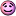## Recommended Posts

I did a google and found out that the square root of i is [sqrt 2]/2 + [sqrt 2]/2i, but the reasoning behind it is stuff way out of my league. Can anybody simplify this to a reasoning easier to understand?

##### Share on other sites

It´s often usefull to write complex numbers a+bi in a polar coordinate way as r*exp(ip) with r and p both being reals. If you draw the complex plane in the usual way, r will be the distance of a+bi from the origin and p will be the counter-clockwise angle of this connection line to the positive Re-axis. The multiplication rule in this representation is z1 * z2 = r1*exp(ip1) *r2*exp(ip2) = (r1*r2) * exp(i(p1+p2) or in other words: Multiply the distances, add the angles.

So for the square root of a complex number z=d*exp(ip) you are looking for a number z' =d' *exp(ip') such that d'*d' = d and p'+p' = p (you have to pay attention that the addition is modulo 2pi, so p/2 is as well a solution for p' as p/2+pi is).

The imaginary number can be written as i = 1*exp(i*pi/2) so d=1 and p=pi/2. From d=1 it follows directly that d'=1 (negative distances are not possible). From p=pi/2 => p' = pi/4 or pi/4+pi.

##### Share on other sites

A simple explaination:

$i$ will be our imaginary constant.

$\sqrt{i} = a + bi$

All solutions can be expressed like that where $a$ and $b$ are real numbers.

$i = a^2 - b^2 + 2abi$

We can break that apart into 2 equations, with the reals in one and the imaginaries in the other, we get:

(I) $0 = a^{2} - b^{2}$

and

(II) $i = 2abi$

(II) $1 = 2ab$

Normal algebraic manipulation with (I) and (II) using quadratic formula and such will conclude that:

$a = \frac{1}{2}$ and $b=\frac{\sqrt{2}}{2}$

Thus $\sqrt{i} = \tfrac{1}{2} + \tfrac{\sqrt{2}}{2}$

##### Share on other sites

• 2 weeks later...
A simple explaination:

$i$ will be our imaginary constant.

$\sqrt{i} = a + bi$

All solutions can be expressed like that where $a$ and $b$ are real numbers.

$i = a^2 - b^2 + 2abi$

We can break that apart into 2 equations' date=' with the reals in one and the imaginaries in the other, we get:

(I) [math'] 0 = a^{2} - b^{2}[/math]

and

(II) $i = 2abi$

(II) $1 = 2ab$

Normal algebraic manipulation with (I) and (II) using quadratic formula and such will conclude that:

$a = \frac{1}{2}$ and $b=\frac{\sqrt{2}}{2}$

Thus $\sqrt{i} = \tfrac{1}{2} + \tfrac{\sqrt{2}}{2}$

I think you mean [imath]a = b = 1/\sqrt{2} [/imath] and [imath]\sqrt{i} = 1/\sqrt{2} + i/\sqrt{2}[/imath]

##### Share on other sites

Ooops... I think my brain farted, thanks for correcting me.

I think instead of $\sqrt{i} = \tfrac{1}{2} + \tfrac{\sqrt{2}}{2}$ I meant $\sqrt{i} = \tfrac{1}{\sqrt{2}} + \tfrac{\sqrt{2}i}{2}$... it was close##### Share on other sites

• 1 month later...

blah. you get two solutions in this example, which are intiutively +/- blah blah. generally when you take a nth root of a complex number it will spit out n values.

but its been so long i am not entirely sure ;_; maybe someone can reassure me.

##### Share on other sites

• 1 year later...
I think you mean [imath]a = b = 1/\sqrt{2} [/imath] and [imath]\sqrt{i} = 1/\sqrt{2} + i/\sqrt{2}[/imath]

Actually there are two solutions also [imath]a = b = -1/\sqrt{2} [/imath]

## Create an account

Register a new account